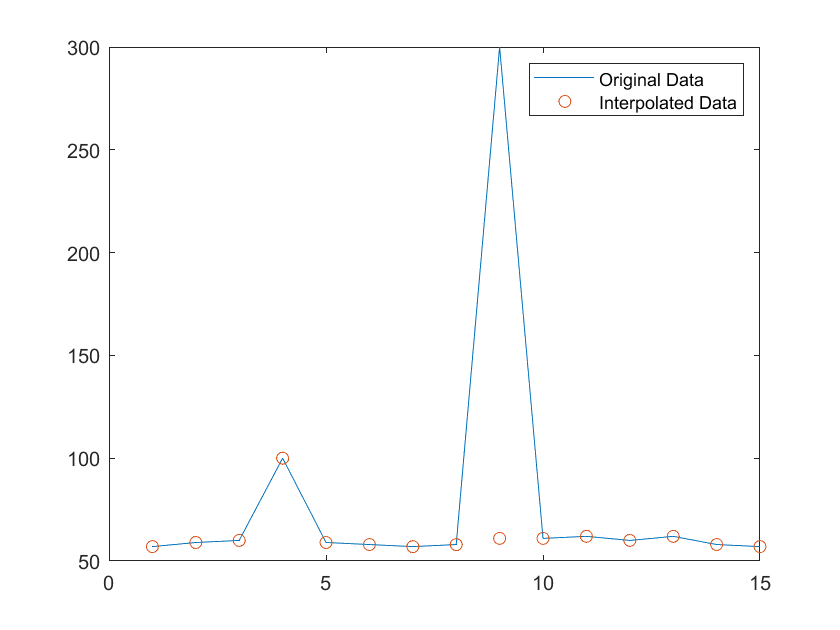# 利用线性插值剔除离群点

``````A = [57 59 60 100 59 58 57 58 300 61 62 60 62 58 57];
B = filloutliers(A,'linear'); % 使用线性插值替代异常点
plot(1:15,A,1:15,B,'o')
legend('Original Data','Interpolated Data')
``````

Create a vector of data containing an outlier, and use linear interpolation to replace the outlier. Plot the original and filled data.‘center’ 使用由 findmethod 决定的中心值进行填充
‘clip’ 对于比 findmethod 决定的下阈值还小的元素，用下阈值填充。对于比 findmethod 决定的上阈值还大的元素，用上阈值填充。
‘previous’ 使用上一个非离群值进行填充
‘next’ 使用下一个非离群值进行填充
‘nearest’ 使用最接近的非离群值进行填充
‘linear’ 使用相邻的非离群值的线性插值进行填充
‘spline’ 使用分段三次样条插值进行填充
‘pchip’ 使用保形分段三次样条插值进行填充

# 利用均值确定离群值

``````A = [57 59 60 100 59 58 57 58 300 61 62 60 62 58 57];
B = filloutliers(A,'nearest','mean');
plot(1:15,A,1:15,B,'o')
legend('Original Data','Interpolated Data')
``````‘mean’ 离群值定义为与均值相差超过三倍标准差的元素。此方法比 ‘median’ 快，但没有它可靠。
‘quartiles’ 离群值定义为比上四分位数 (75%) 大 1.5 个四分位差以上或比下四分位数 (25%) 小 1.5 个四分位差以上的元素。当 A 中的数据不是正态分布时，此方法很有用。
‘grubbs’ 使用 Grubbs 检验检测离群值，并基于假设检验每次迭代删除一个离群值。此方法假设 A 中的数据呈正态分布。
‘gesd’ 使用广义极端 Student 偏差检验检测离群值。此迭代方法与 ‘grubbs’ 类似，但当有多个离群值互相遮盖时，此方法的执行效果更好。

# 利用移动窗口确定离群值

``````x = -2*pi:0.1:2*pi;
A = sin(x);
A(47) = 0;
``````

``````t = datetime(2017,1,1,0,0,0) + hours(0:length(x)-1);
``````

``````[B,TF,U,L,C] = filloutliers(A,'clip','movmedian',hours(5),'SamplePoints',t);
plot(t,A,t,B,'o')
legend('Original Data','Filled Data')
``````

``````L(TF)
``````
``````ans = -0.8779
``````‘movmedian’ 离群值定义为在 window 指定的窗口长度内，与局部中位数相差超过三倍局部换算 MAD 的元素。
‘movmean’ 离群值定义为在 window 指定的窗口长度内，与局部均值相差超过三倍局部标准差的元素。

# 离群点补偿阈值设置

Find the outlier in a vector of data, and replace it using the ‘clip’ method. Plot the original data, the filled data, and the thresholds and center value determined by the detection method. ‘clip’ replaces the outlier with the upper threshold value.

``````A = [60 59 49 49 58 100 61 57 48 58 200 0 500 41];
x = 1:length(A);
[B,TF,lower,upper,center] = filloutliers(A,'clip');
plot(x,A,x,B,'o',x,lower*ones(1,length(A)),x,upper*ones(1,length(A)),x,center*ones(1,length(A)))
legend('Original Data','Filled Data','Lower Threshold','Upper Threshold','Center Value')
``````